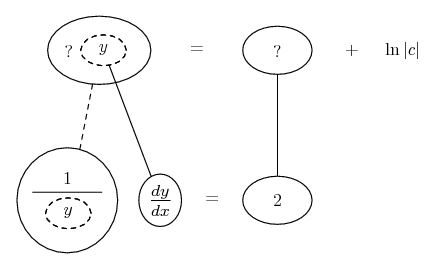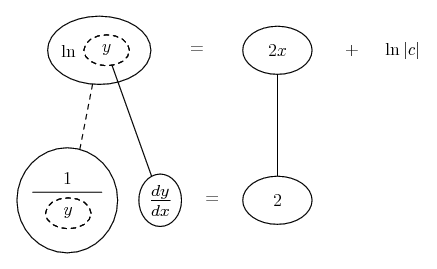# Thread: Simple curve equation help

1. ## Simple curve equation help

A curve passes through the point (0, 9) and has the property that the slope of the curve at every point P is twice the y-coordinate of P. What is the equation of the curve?

2. Have you formed an equation involving the slope (dy/dx) and y?

(Don't worry yet about (0,9).)

It's a differential equation... separate variables

Edit:

Just in case a picture helps...... where... is the chain rule. Straight continuous lines differentiate downwards (integrate up) with respect to x, and the straight dashed line similarly but with respect to the dashed balloon expression (the inner function of the composite which is subject to the chain rule).

Complete separation of variables (which some will prefer) uses the chain rule to split dy/dx into differentials, one each side, so you can think Riemann sums when integrating. This way here, though, you just think: anti-differentiate each side with respect to x. The chain rule means you also anti-diff 1/y with respect to y.

Let's also call the constant of integration ln|c| instead of the usual c, to help things along slightly when we take the exp of each side of the top equation (when we've got one).

Then you'll be ready to use (0,9).
__________________________________________

Don't integrate - balloontegrate!

http://www.ballooncalculus.org/examples/gallery.html

http://www.ballooncalculus.org/asy/doc.html

3. i'm still pretty lost...

so the equation is y=ln|x|+x^2+9 anywhere close to being right? It's obviously not. But I'm getting warmer.

forgive my ignorance. I'm a freshman.

4. Not sure what you did there... y = mx + c, maybe? Anyway, what happens here is we start with a differential equation

$\displaystyle \frac{dy}{dx} = 2y$

This is called 'separable' because we can get all the y's over on the left along the bottom here...Then integrate both sides of the equation (with respect to x, but using the chain rule)...Now take the exp of each side of the top row and simplify the right hand side...

... and THEN use (0,9), to find c!

__________________________________________

Don't integrate - balloontegrate!

Balloon Calculus: Gallery

Balloon Calculus Drawing with LaTeX and Asymptote!

#### Search Tags

curve, equation, simple